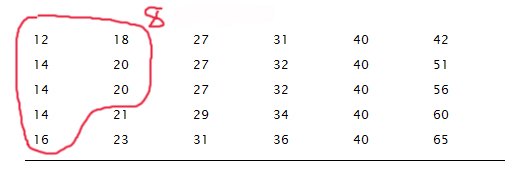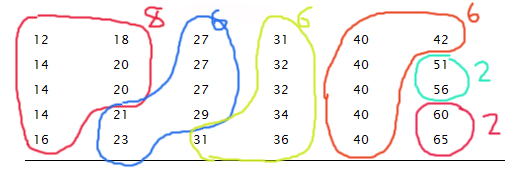# Making Frequency Distributions and Histograms by Hand

Generally, statisticians (and any sane person) will use some kind of statistical program like R or minitab to make their statistical graphs. However, it is still surprisingly common to see textbooks do everything by hand and in the end, learning how to make a histogram by hand is a great way to get better at reading them and figuring out what the problem is when a computer or calculator gives you something you don’t expect. In this lesson, we will look at the step-by-step process of making a frequency distribution and a histogram.

To show you how to do this, we will be using the data set below. I went ahead and put the numbers in order which will make everything much easier.

 12 14 14 14 16 18 20 20 21 23 27 27 27 29 31 31 32 32 34 36 40 40 40 40 40 42 51 56 60 65

To make a histogram by hand, we must first find the frequency distribution. The idea behind a frequency distribution is to break the data into groups (called classes or bins) so that we can better see patterns. It is sort of like the difference between asking you your age and asking you if you are between 20 and 25. In the second question, I am grouping up the ages. This way if I have a HUGE data set (like many are) I can see the patterns (like are most people older or younger) much easier than if I just tried to decipher a large list of numbers.

## Steps to Making Your Frequency Distribution

### Step 1: Calculate the range of the data set

The range is the difference between the largest value and the smallest value. We need this to figure out how much “space” we need to divide into groups. In this example:

$$\text{Range}=65-12=53$$

### Step 2: Divide the range by the number of groups you want and then round up

Doing this allows us to figure out how large each group is. It’s as if we are going to cut a board into equal pieces. In step 1, we measured how long the board is and now we are deciding how big each piece will be.

Hmmm… but how many groups to have? Too many, and our graphs and tables won’t be much better than a list of numbers. Too few, and the pattern will be hidden with too little detail. Often, a good number of groups is 5 or 6 although there are some rules that people use to decide this. MORE OFTEN, people will let the computer decide and then adjust if they want to while textbooks will tell you how many groups to use. But if you are working with the dataset yourself, you will have to see what the graph looks like before you can be sure you chose a good number.

Let’s say that we choose to have 6 groups. If we do this then:

$$\dfrac{53}{6}=8.8$$

The number we just found is commonly called the class width. We will round this up to 9 just because it is easier to work with that way. A computer would probably keep the 8.8 so be aware that sometimes you will see this number as a decimal. NOTE: In general, people who are doing this by hand always round up even if it was 8.1!

### Step 3: Use the class width to create your groups

I’m going to start at the smallest number we have, which is 12, and count by 9 until I have my 6 groups. For example, my first group will be 12 to 21 since 12+9=21. My next group will be 21-30 since 21+9=30… and so on. I’ll put these in a table and label them “classes”. I will also add “frequency” to the table.:

Classes Frequency
12 – 21
21 – 30
30 – 39
39 – 48
48 – 57
57 – 66

### Step 4: Find the frequency for each group

This part is probably the most tedious and the main reason why it is unrealistic to make a frequency distribution or histogram by hand for a very large data set. We are going to count how many points are in each group. Let’s start with our first group: 12 – 21. We want to count how many points are between 12 and 21 NOT INCLUDING 21. You see the overlap between the groups right? That’s to account for decimals and we keep it even when we don’t have any. The right hand endpoint of any group isn’t included in that group. It goes in the next group. That means 21 would be in the second group and any 30 we have would be counted in the third group.

Back to the first group: 12-21. I have circled the points which would be included in this group:Alright – now I update the table with this information!

Classes Frequency
12 – 21 8
21 – 30
30 – 39
39 – 48
48 – 57
57 – 66

Continuing with this pattern (each group is a different color!):Classes Frequency
12 – 21 8
21 – 30 6
30 – 39 6
39 – 48 6
48 – 57 2
57 – 66 2

That last table is our frequency distribution! To make a histogram from this, we will use the groups on the horizontal axis and the frequency on the vertical axis. Finally, we will use bars to represent the the frequency of each individual group. With this data, the finished histogram will look like the one below.You can see another example of how this is done in the video below.